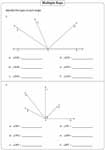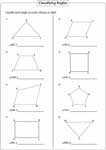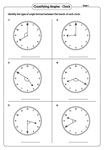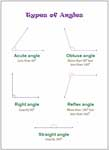# Classifying and Identifying Angles Worksheets

Based on the measurement, the angles can be broadly classified as acute, right, obtuse and reflex. This page contains worksheets in identifying types, classifying angles, measure and classify, drawing examples according to the given type, identifying types from multiple rays, classifying angles in geometric shapes, types of angles formed by clocks, counting types and classification charts.

Classifying Angles

Each worksheet has 12 problems classifying types of angles. Level 1 has acute, right, obtuse and straight angles. Level 2 has all angles in level 1 in addition to reflex angle.Level 1:

Level 2:

Measure and Classify

Measure each angle using protractor and identify the type.Level 1:

Level 2:

Drawing Angles

Read the type and draw the angle. Students should set up their own example for each angle-type.Level 1:

Level 2:

Identifying Angles: Multiple Rays

There are two or more rays in each problem. Read the angle representation and identify the types.Geometric Shapes

A geometric shape and an angle are given in each problem. Identify the type of each angle.Hands of a Clock

Identify the type of angle formed between a pair of hands in each clock.Counting Types

Each closed shape has either acute, obtuse, right or all the angles. Count the types of angles formed in each figure.Angle Classification Charts

Each chart displays the classification of angles. Download the charts for learning or teaching purposes.The entire collection of worksheets on classifying and identifying angles can be downloaded in a jiffy!

Related Worksheets